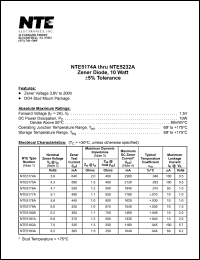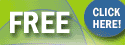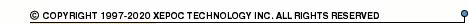More than4 307 195 components listedNTE5174 series datasheets. Manufacturer: NTE.

 NTE5174A Zener diode, 10 watt, +-5% tolerance. Nominal zener voltage Vz = 3.9V. Zener test current Izt = 640mA. in 2-pin DO4 package. Operational temperature range from -65°C to 175°C. Datasheet*) NTE5175A Zener diode, 10 watt, +-5% tolerance. Nominal zener voltage Vz = 4.3V. Zener test current Izt = 580mA. in 2-pin DO4 package. Operational temperature range from -65°C to 175°C. Datasheet*) NTE5176A Zener diode, 10 watt, +-5% tolerance. Nominal zener voltage Vz = 4.7V. Zener test current Izt = 530mA. in 2-pin DO4 package. Operational temperature range from -65°C to 175°C. Datasheet*) NTE5177A Zener diode, 10 watt, +-5% tolerance. Nominal zener voltage Vz = 5.1V. Zener test current Izt = 490mA. in 2-pin DO4 package. Operational temperature range from -65°C to 175°C. Datasheet*) NTE5178A Zener diode, 10 watt, +-5% tolerance. Nominal zener voltage Vz = 5.6V. Zener test current Izt = 445mA. in 2-pin DO4 package. Operational temperature range from -65°C to 175°C. Datasheet*) NTE5179A Zener diode, 10 watt, +-5% tolerance. Nominal zener voltage Vz = 6.0V. Zener test current Izt = 425mA. in 2-pin DO4 package. Operational temperature range from -65°C to 175°C. Datasheet*) NTE5180A Zener diode, 10 watt, +-5% tolerance. Nominal zener voltage Vz = 6.2V. Zener test current Izt = 405mA. in 2-pin DO4 package. Operational temperature range from -65°C to 175°C. Datasheet*) NTE5181A Zener diode, 10 watt, +-5% tolerance. Nominal zener voltage Vz = 6.8V. Zener test current Izt = 370mA. in 2-pin DO4 package. Operational temperature range from -65°C to 175°C. Datasheet*) NTE5182A Zener diode, 10 watt, +-5% tolerance. Nominal zener voltage Vz = 7.5V. Zener test current Izt = 335mA. in 2-pin DO4 package. Operational temperature range from -65°C to 175°C. Datasheet*) NTE5183A Zener diode, 10 watt, +-5% tolerance. Nominal zener voltage Vz = 8.2V. Zener test current Izt = 305mA. in 2-pin DO4 package. Operational temperature range from -65°C to 175°C. Datasheet*) NTE5184A Zener diode, 10 watt, +-5% tolerance. Nominal zener voltage Vz = 8.7V. Zener test current Izt = 290mA. in 2-pin DO4 package. Operational temperature range from -65°C to 175°C. Datasheet*) NTE5185A Zener diode, 10 watt, +-5% tolerance. Nominal zener voltage Vz = 9.1V. Zener test current Izt = 275mA. in 2-pin DO4 package. Operational temperature range from -65°C to 175°C. Datasheet*) NTE5186A Zener diode, 10 watt, +-5% tolerance. Nominal zener voltage Vz = 10V. Zener test current Izt = 250mA. in 2-pin DO4 package. Operational temperature range from -65°C to 175°C. Datasheet*) NTE5187A Zener diode, 10 watt, +-5% tolerance. Nominal zener voltage Vz = 11V. Zener test current Izt = 230mA. in 2-pin DO4 package. Operational temperature range from -65°C to 175°C. Datasheet*) NTE5188A Zener diode, 10 watt, +-5% tolerance. Nominal zener voltage Vz = 12V. Zener test current Izt = 210mA. in 2-pin DO4 package. Operational temperature range from -65°C to 175°C. Datasheet*) NTE5189A Zener diode, 10 watt, +-5% tolerance. Nominal zener voltage Vz = 13V. Zener test current Izt = 190mA. in 2-pin DO4 package. Operational temperature range from -65°C to 175°C. Datasheet*) NTE5190A Zener diode, 10 watt, +-5% tolerance. Nominal zener voltage Vz = 14V. Zener test current Izt = 180mA. in 2-pin DO4 package. Operational temperature range from -65°C to 175°C. Datasheet*) NTE5191A Zener diode, 10 watt, +-5% tolerance. Nominal zener voltage Vz = 15V. Zener test current Izt = 170mA. in 2-pin DO4 package. Operational temperature range from -65°C to 175°C. Datasheet*) NTE5192A Zener diode, 10 watt, +-5% tolerance. Nominal zener voltage Vz = 16V. Zener test current Izt = 155mA. in 2-pin DO4 package. Operational temperature range from -65°C to 175°C. Datasheet*) NTE5193A Zener diode, 10 watt, +-5% tolerance. Nominal zener voltage Vz = 17V. Zener test current Izt = 145mA. in 2-pin DO4 package. Operational temperature range from -65°C to 175°C. Datasheet*) NTE5194A Zener diode, 10 watt, +-5% tolerance. Nominal zener voltage Vz = 18V. Zener test current Izt = 140mA. in 2-pin DO4 package. Operational temperature range from -65°C to 175°C. Datasheet*) NTE5195A Zener diode, 10 watt, +-5% tolerance. Nominal zener voltage Vz = 19V. Zener test current Izt = 130mA. in 2-pin DO4 package. Operational temperature range from -65°C to 175°C. Datasheet*) NTE5196A Zener diode, 10 watt, +-5% tolerance. Nominal zener voltage Vz = 20V. Zener test current Izt = 125mA. in 2-pin DO4 package. Operational temperature range from -65°C to 175°C. Datasheet*) NTE5197A Zener diode, 10 watt, +-5% tolerance. Nominal zener voltage Vz = 22V. Zener test current Izt = 115mA. in 2-pin DO4 package. Operational temperature range from -65°C to 175°C. Datasheet*) NTE5198A Zener diode, 10 watt, +-5% tolerance. Nominal zener voltage Vz = 24V. Zener test current Izt = 105mA. in 2-pin DO4 package. Operational temperature range from -65°C to 175°C. Datasheet*) NTE5199A Zener diode, 10 watt, +-5% tolerance. Nominal zener voltage Vz = 25V. Zener test current Izt = 100mA. in 2-pin DO4 package. Operational temperature range from -65°C to 175°C. Datasheet*) NTE5200A Zener diode, 10 watt, +-5% tolerance. Nominal zener voltage Vz = 27V. Zener test current Izt = 95mA. in 2-pin DO4 package. Operational temperature range from -65°C to 175°C. Datasheet*) NTE5201A Zener diode, 10 watt, +-5% tolerance. Nominal zener voltage Vz = 28V. Zener test current Izt = 90mA. in 2-pin DO4 package. Operational temperature range from -65°C to 175°C. Datasheet*) NTE5202A Zener diode, 10 watt, +-5% tolerance. Nominal zener voltage Vz = 30V. Zener test current Izt = 85mA. in 2-pin DO4 package. Operational temperature range from -65°C to 175°C. Datasheet*) NTE5203A Zener diode, 10 watt, +-5% tolerance. Nominal zener voltage Vz = 33V. Zener test current Izt = 75mA. in 2-pin DO4 package. Operational temperature range from -65°C to 175°C. Datasheet*) NTE5204A Zener diode, 10 watt, +-5% tolerance. Nominal zener voltage Vz = 36V. Zener test current Izt = 70mA. in 2-pin DO4 package. Operational temperature range from -65°C to 175°C. Datasheet*) NTE5205A Zener diode, 10 watt, +-5% tolerance. Nominal zener voltage Vz = 39V. Zener test current Izt = 65mA. in 2-pin DO4 package. Operational temperature range from -65°C to 175°C. Datasheet*) NTE5206A Zener diode, 10 watt, +-5% tolerance. Nominal zener voltage Vz = 43V. Zener test current Izt = 60mA. in 2-pin DO4 package. Operational temperature range from -65°C to 175°C. Datasheet*) NTE5207A Zener diode, 10 watt, +-5% tolerance. Nominal zener voltage Vz = 45V. Zener test current Izt = 55mA. in 2-pin DO4 package. Operational temperature range from -65°C to 175°C. Datasheet*) NTE5208A Zener diode, 10 watt, +-5% tolerance. Nominal zener voltage Vz = 47V. Zener test current Izt = 55mA. in 2-pin DO4 package. Operational temperature range from -65°C to 175°C. Datasheet*) NTE5209A Zener diode, 10 watt, +-5% tolerance. Nominal zener voltage Vz = 50V. Zener test current Izt = 50mA. in 2-pin DO4 package. Operational temperature range from -65°C to 175°C. Datasheet*) NTE5210A Zener diode, 10 watt, +-5% tolerance. Nominal zener voltage Vz = 51V. Zener test current Izt = 50mA. in 2-pin DO4 package. Operational temperature range from -65°C to 175°C. Datasheet*) NTE5211A Zener diode, 10 watt, +-5% tolerance. Nominal zener voltage Vz = 52V. Zener test current Izt = 50mA. in 2-pin DO4 package. Operational temperature range from -65°C to 175°C. Datasheet*) NTE5212A Zener diode, 10 watt, +-5% tolerance. Nominal zener voltage Vz = 56V. Zener test current Izt = 45mA. in 2-pin DO4 package. Operational temperature range from -65°C to 175°C. Datasheet*) NTE5213A Zener diode, 10 watt, +-5% tolerance. Nominal zener voltage Vz = 60V. Zener test current Izt = 43mA. in 2-pin DO4 package. Operational temperature range from -65°C to 175°C. Datasheet*) NTE5214A Zener diode, 10 watt, +-5% tolerance. Nominal zener voltage Vz = 62V. Zener test current Izt = 40mA. in 2-pin DO4 package. Operational temperature range from -65°C to 175°C. Datasheet*) NTE5215A Zener diode, 10 watt, +-5% tolerance. Nominal zener voltage Vz = 68V. Zener test current Izt = 37mA. in 2-pin DO4 package. Operational temperature range from -65°C to 175°C. Datasheet*) NTE5216A Zener diode, 10 watt, +-5% tolerance. Nominal zener voltage Vz = 75V. Zener test current Izt = 33mA. in 2-pin DO4 package. Operational temperature range from -65°C to 175°C. Datasheet*) NTE5217A Zener diode, 10 watt, +-5% tolerance. Nominal zener voltage Vz = 82V. Zener test current Izt = 30mA. in 2-pin DO4 package. Operational temperature range from -65°C to 175°C. Datasheet*) NTE5218A Zener diode, 10 watt, +-5% tolerance. Nominal zener voltage Vz = 87V. Zener test current Izt = 29mA. in 2-pin DO4 package. Operational temperature range from -65°C to 175°C. Datasheet*) NTE5219A Zener diode, 10 watt, +-5% tolerance. Nominal zener voltage Vz = 91V. Zener test current Izt = 28mA. in 2-pin DO4 package. Operational temperature range from -65°C to 175°C. Datasheet*) NTE5220A Zener diode, 10 watt, +-5% tolerance. Nominal zener voltage Vz = 100V. Zener test current Izt = 25mA. in 2-pin DO4 package. Operational temperature range from -65°C to 175°C. Datasheet*) NTE5221A Zener diode, 10 watt, +-5% tolerance. Nominal zener voltage Vz = 105V. Zener test current Izt = 25mA. in 2-pin DO4 package. Operational temperature range from -65°C to 175°C. Datasheet*) NTE5222A Zener diode, 10 watt, +-5% tolerance. Nominal zener voltage Vz = 110V. Zener test current Izt = 23mA. in 2-pin DO4 package. Operational temperature range from -65°C to 175°C. Datasheet*) NTE5223A Zener diode, 10 watt, +-5% tolerance. Nominal zener voltage Vz = 120V. Zener test current Izt = 20mA. in 2-pin DO4 package. Operational temperature range from -65°C to 175°C. Datasheet*) NTE5224A Zener diode, 10 watt, +-5% tolerance. Nominal zener voltage Vz = 130V. Zener test current Izt = 19mA. in 2-pin DO4 package. Operational temperature range from -65°C to 175°C. Datasheet*) NTE5225A Zener diode, 10 watt, +-5% tolerance. Nominal zener voltage Vz = 140V. Zener test current Izt = 18mA. in 2-pin DO4 package. Operational temperature range from -65°C to 175°C. Datasheet*) NTE5226A Zener diode, 10 watt, +-5% tolerance. Nominal zener voltage Vz = 150V. Zener test current Izt = 17mA. in 2-pin DO4 package. Operational temperature range from -65°C to 175°C. Datasheet*) NTE5227A Zener diode, 10 watt, +-5% tolerance. Nominal zener voltage Vz = 160V. Zener test current Izt = 16mA. in 2-pin DO4 package. Operational temperature range from -65°C to 175°C. Datasheet*) NTE5228A Zener diode, 10 watt, +-5% tolerance. Nominal zener voltage Vz = 170V. Zener test current Izt = 15mA. in 2-pin DO4 package. Operational temperature range from -65°C to 175°C. Datasheet*) NTE5229A Zener diode, 10 watt, +-5% tolerance. Nominal zener voltage Vz = 175V. Zener test current Izt = 14mA. in 2-pin DO4 package. Operational temperature range from -65°C to 175°C. Datasheet*) NTE5230A Zener diode, 10 watt, +-5% tolerance. Nominal zener voltage Vz = 180V. Zener test current Izt = 14mA. in 2-pin DO4 package. Operational temperature range from -65°C to 175°C. Datasheet*) NTE5231A Zener diode, 10 watt, +-5% tolerance. Nominal zener voltage Vz = 190V. Zener test current Izt = 13mA. in 2-pin DO4 package. Operational temperature range from -65°C to 175°C. Datasheet*) NTE5232A Zener diode, 10 watt, +-5% tolerance. Nominal zener voltage Vz = 200V. Zener test current Izt = 12mA. in 2-pin DO4 package. Operational temperature range from -65°C to 175°C. Datasheet*) NTE5174AK 3.9V, 10Wt General purpose voltage reference/regulator diode Datasheet*) NTE5175AK 4.3V, 10Wt General purpose voltage reference/regulator diode Datasheet*) NTE5176AK 4.7V, 10Wt General purpose voltage reference/regulator diode Datasheet*) NTE5177AK 5.1V, 10Wt General purpose voltage reference/regulator diode Datasheet*) NTE5178AK 5.6V, 10Wt General purpose voltage reference/regulator diode Datasheet*) NTE5179AK 6.0V, 10Wt General purpose voltage reference/regulator diode Datasheet*) NTE5180AK 6.2V, 10Wt General purpose voltage reference/regulator diode Datasheet*) NTE5181AK 6.8V, 10Wt General purpose voltage reference/regulator diode Datasheet*) NTE5182AK 7.5V, 10Wt General purpose voltage reference/regulator diode Datasheet*) NTE5183AK 8.2V, 10Wt General purpose voltage reference/regulator diode Datasheet*) NTE5184AK 8.7V, 10Wt General purpose voltage reference/regulator diode Datasheet*) NTE5185AK 9.1V, 10Wt General purpose voltage reference/regulator diode Datasheet*) NTE5186AK 10.0V, 10Wt General purpose voltage reference/regulator diode Datasheet*) NTE5187AK 11.0V, 10Wt General purpose voltage reference/regulator diode Datasheet*) NTE5188AK 12.0V, 10Wt General purpose voltage reference/regulator diode Datasheet*) NTE5189AK 13.0V, 10Wt General purpose voltage reference/regulator diode Datasheet*) NTE5190AK 14.0V, 10Wt General purpose voltage reference/regulator diode Datasheet*) NTE5191AK 15.0V, 10Wt General purpose voltage reference/regulator diode Datasheet*) NTE5192AK 16.0V, 10Wt General purpose voltage reference/regulator diode Datasheet*) NTE5193AK 17.0V, 10Wt General purpose voltage reference/regulator diode Datasheet*) NTE5194AK 18.0V, 10Wt General purpose voltage reference/regulator diode Datasheet*) NTE5195AK 19.0V, 10Wt General purpose voltage reference/regulator diode Datasheet*) NTE5196AK 20.0V, 10Wt General purpose voltage reference/regulator diode Datasheet*) NTE5197AK 22.0V, 10Wt General purpose voltage reference/regulator diode Datasheet*) NTE5198AK 24.0V, 10Wt General purpose voltage reference/regulator diode Datasheet*) NTE5199AK 25.0V, 10Wt General purpose voltage reference/regulator diode Datasheet*) NTE5200AK 27.0V, 10Wt General purpose voltage reference/regulator diode Datasheet*) NTE5201AK 28.0V, 10Wt General purpose voltage reference/regulator diode Datasheet*) NTE5202AK 30.0V, 10Wt General purpose voltage reference/regulator diode Datasheet*) NTE5203AK 33.0V, 10Wt General purpose voltage reference/regulator diode Datasheet*) NTE5204AK 36.0V, 10Wt General purpose voltage reference/regulator diode Datasheet*) NTE5205AK 39.0V, 10Wt General purpose voltage reference/regulator diode Datasheet*) NTE5206AK 43.0V, 10Wt General purpose voltage reference/regulator diode Datasheet*) NTE5207AK 45.0V, 10Wt General purpose voltage reference/regulator diode Datasheet*) NTE5208AK 47.0V, 10Wt General purpose voltage reference/regulator diode Datasheet*) NTE5209AK 50.0V, 10Wt General purpose voltage reference/regulator diode Datasheet*) NTE5210AK 51.0V, 10Wt General purpose voltage reference/regulator diode Datasheet*) NTE5211AK 52.0V, 10Wt General purpose voltage reference/regulator diode Datasheet*) NTE5212AK 56.0V, 10Wt General purpose voltage reference/regulator diode Datasheet*) NTE5213AK 60.0V, 10Wt General purpose voltage reference/regulator diode Datasheet*) NTE5214AK 62.0V, 10Wt General purpose voltage reference/regulator diode Datasheet*) NTE5215AK 68.0V, 10Wt General purpose voltage reference/regulator diode Datasheet*) NTE5216AK 75.0V, 10Wt General purpose voltage reference/regulator diode Datasheet*) NTE5217AK 82.0V, 10Wt General purpose voltage reference/regulator diode Datasheet*) NTE5218AK 87.0V, 10Wt General purpose voltage reference/regulator diode Datasheet*) NTE5219AK 91.0V, 10Wt General purpose voltage reference/regulator diode Datasheet*) NTE5220AK 100V, 10Wt General purpose voltage reference/regulator diode Datasheet*) NTE5221AK 105V, 10Wt General purpose voltage reference/regulator diode Datasheet*) NTE5222AK 110V, 10Wt General purpose voltage reference/regulator diode Datasheet*) NTE5223AK 120V, 10Wt General purpose voltage reference/regulator diode Datasheet*) NTE5224AK 130V, 10Wt General purpose voltage reference/regulator diode Datasheet*) NTE5225AK 140V, 10Wt General purpose voltage reference/regulator diode Datasheet*) NTE5226AK 150V, 10Wt General purpose voltage reference/regulator diode Datasheet*) NTE5227AK 160V, 10Wt General purpose voltage reference/regulator diode Datasheet*) NTE5228AK 170V, 10Wt General purpose voltage reference/regulator diode Datasheet*) NTE5229AK 175V, 10Wt General purpose voltage reference/regulator diode Datasheet*) NTE5230AK 180V, 10Wt General purpose voltage reference/regulator diode Datasheet*) NTE5231AK 190V, 10Wt General purpose voltage reference/regulator diode Datasheet*) NTE5232AK 200V, 10Wt General purpose voltage reference/regulator diode Datasheet*)Industrial Laser Solutions for Manufacturing Magazine.Subscribe now for FREE !